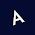# Quiz Diva Spot the Different Food Quiz Answers Video

Q 1. Which image is different?

Options
A
B
C
D

Q 2. Which image is different?

Options
A
B
C
D

Q 3. Which image is different?

Options
A
B
C
D

Q 4. Which image is different?

Options
A
B
C
D

Q 5. Which image is different?

Options
A
B
C
D

Q 6. Which image is different?

Options
A
B
C
D

Q 7. Which image is different?

Options
A
B
C
D

Q 8. Which image is different?

Options
A
B
C
D

Q 9. Which image is different?

Options
A
B
C
D

Q 10. Which image is different?

Options
A
B
C
D

Q 11. Which image is different?

Options
A
B
C
D

Q 12. Which image is different?

Options
A
B
C
D

Q 13. Which image is different?

Options
A
B
C
D

Q 14. Which image is different?

Options
A
B
C
D

Q 15. Which image is different?

Options
A
B
C
D

Q 16. Which image is different?

Options
A
B
C
D

Q 17. Which image is different?

Options
A
B
C
D

Q 18. Which image is different?

Options
A
B
C
D

Q 19. Which image is different?

Options
A
B
C
D

Q 20. Which image is different?

Options
A
B
C
D

Q 21. Which image is different?

Options
A
B
C
D

Q 22. Which image is different?

Options
A
B
C
D

Q 23. Which image is different?

Options
A
B
C
D

Q 24. Which image is different?

Options
A
B
C
D

Q 25. Which image is different?

Options
A
B
C
D

Q 26. Which image is different?

Options
A
B
C
D

Q 27. Which image is different?

Options
A
B
C
D

Q 28. Which image is different?

Options
A
B
C
D

Q 29. Which image is different?

Options
A
B
C
D

Q 30. Which image is different?

Options
A
B
C
D

Q 31. Which image is different?

Options
A
B
C
D

Q 32. Which image is different?

Options
A
B
C
D

Q 33. Which image is different?

Options
A
B
C
D

Q 34. Which image is different?

Options
A
B
C
D

Q 35. Which image is different?

Options
A
B
C
D

Q 36. Which image is different?

Options
A
B
C
D

Q 37. Which image is different?

Options
A
B
C
D

Q 38. Which image is different?

Options
A
B
C
D

Q 39. Which image is different?

Options
A
B
C
D

Q 40. Which image is different?

Options
A
B
C
D

Q 41. Which image is different?

Q 42. Which image is different?
Q 43. Which image is different?

Q 44. Which image is different?

Q 45. Which image is different?

Options

A
B
C
D

Q 46. Which image is different?

Options

A
B
C
D

Thank you for reading Quiz Diva Spot the Different Food Quiz Answers on MyNeo.

1.Thank you it helped!

2.3.Este site é incrivel

1.2.4.thanks for letting me earn 2 robux!

1.all the best.

5.1.Welcome :3 uwu

6.I LOVE DISSSSSSS eeek :33333

7.8.9.Thank you.......

10.Thank u sooooo much

11.thanks so much i redeemed my 100000 robux!!!!

12.This really help to me.

13.this is so helpful

14.I SCORED 97% UGH

15.omg thanks soooooooo much now i can get my 100 mil robux

16.Thank u sir....